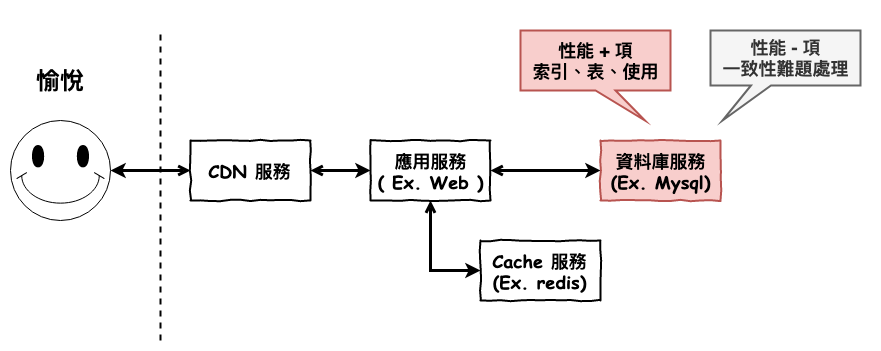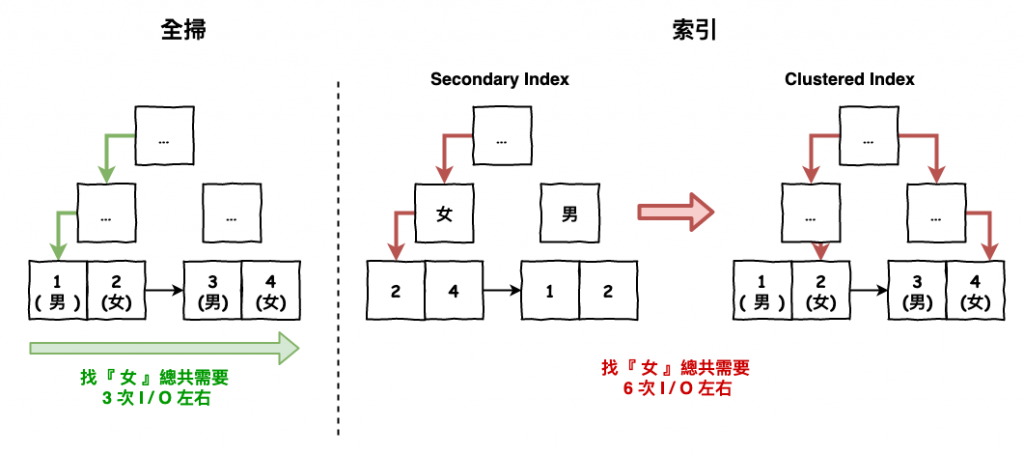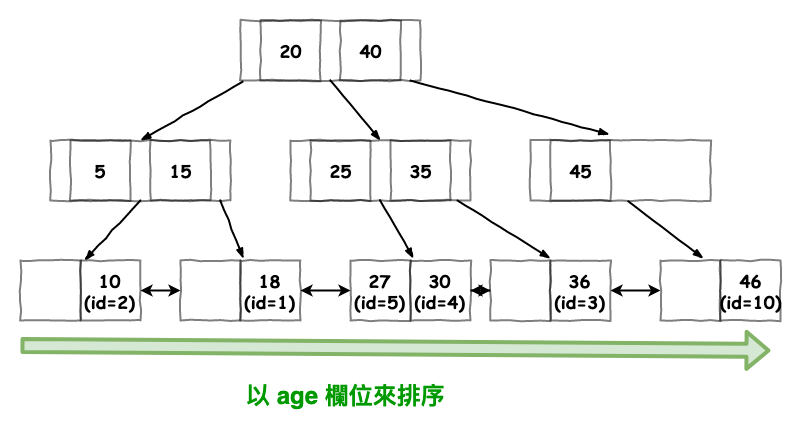#DAY 13
4
Software Development

## 30-13 之資料庫層的優化 - 索引設計與雷區

### 黑色好看版 - 傳送門• 索引的重要小觀念
• 索引的設計流程
• 索引的使用注意事項
• SQL 地雷區

## 索引的重要小觀念

#### 觀念 1 : 不是索引越多越好

• 每個索引都會建立一顆 b+ 樹。
• 每次新增、更新資料時都會改變 b+ 樹。

#### 觀念 2. 懂的使用 Explain 來分析你的 SQL 索引性能解析

explain 這個指令可以讓你知道你下的 sql 語句是否有命中索引。

``````EXPLAIN SELECT * FROM user_no WHERE name = 'mark';

=====================================================

id: 1
select_type: SIMPLE
table: user
partitions: NULL
type: const
possible_keys: PRIMARY
key: PRIMARY
key_len: 8
ref: const
rows: 1
filtered: 100.00
Extra: NULL
1 row in set, 1 warning (0.00 sec)
``````

#### 觀念 3. 索引不是建了就會使用

``````table : user
field: name, sex
index: {sex}
``````

``````SELECT * FROM user WHERE sex = 0 ( 0 代表女生 )
``````

## 索引的設計流程

#### Step 2. 看看候選欄位的基數大小``````SELECT COUNT(DISTINCT {field}/COUNT(*))
``````

``````2/1000 = 0.002
``````

#### Step 3. 決定使用的索引類型

• 一般索引(覆蓋索引) : 在一些常用單獨 query，如果常常需要與其它欄位一起查詢，可考慮建連合索引。
• 連合索引 : 常常需要與其它欄位一起查詢，適合建立。但是要注意先後順序，基本最常查詢的與基數高的適合放最左邊。
• 前綴索引 : 這種大致上用在如果要針對某段文字進行前綴搜尋的情況。
• 唯一索引 : 這種適合在此欄位放貨物編號或啥這種編號的，它可以順到幫你檢查是不是唯一。

## 索引的使用注意事項

### 注意事項 1 : 連合索引的欄位順序

``````{a, b, c}
＝
{a}
{a,b}
{a,b,c}
``````

``````SELECT * FROM Table WHERE a = ? ( good 索引 )
SELECT * FROM Table WHERE a = ? AND b = ? ( good 索引 )
SELECT * FROM Table WHERE b = ? AND a = ? ( good 索引 )

SELECT * FROM Table WHERE b = ? ( bad 全掃 )
SELECT * FROM Table WHERE c = ? ( bad 全掃 )
SELECT * FROM Table WHERE b = ? AND c = ? ( bad 全掃 )
``````

### 注意事項 2 : 儘可能使用索引的排序``````索引欄位: { age }

SELECT * FROM user WHERE age <= 30 ORDER BY age; ( good 索引 )

SELECT * FROM user WHERE age <= 30 ORDER BY name; ( bad using filesort )
``````

#### 那連合索引呢 ?

``````{ age, name }
``````### 注意事項 3 : 有時太多索引，反而會讓優化器混亂

``````a、b、c
a、c、c
b、c、a
b、a、c
c、a、b
c、b、a
``````

## SQL 地雷區

#### 雷區 1 : 使用 != 、 <>、NOT 查詢會變全掃

``````SELECT * FROM user WHERE age != 20 ( bad 全掃 )
SELECT * FROM user WHERE age <> 20 ( bad 全掃 )
SELECT * FROM user WHERE age NOT IN(20) ( bad 全掃 )
``````

#### 雷區 2 : 用 like 且 % 在前面有索引也會變全掃

``````SELECT * FROM user WHERE name like '%-Mark' ( bad 全掃 )
``````

#### 雷區 3 : 誤用 or 時會變全掃

``````索引欄位: {age}

SELECT * FROM user WHERE age = 18 OR name = 'C-Ian'; ( bad 全掃 )

SELECT * FROM user WHERE age = 18 OR name = 'C-Ian'; ( good 索引 )
``````

``````索引欄位: {age}

SELECT * FROM user WHERE age = 18 AND name = 'C-Ian'; ( good 索引 )
``````

#### 雷區 4. 在 WHERE 欄位進行運算

``````索引欄位: {age}

SELECT * FROM user WHERE age/2 = 18; ( bad 全掃 )

SELECT * FROM user WHERE age = 18*2; ( good 索引 )
``````

#### 雷區 5 : 使用一些函數

``````SELECT * FROM test.user where age >= RAND(); ( bad 全掃 )
``````

#### 雷區 6 : SELECT * FROM

clustered Index 抓資料。

``````索引欄位: {name}

SELECT * FROM user; ( bad 會走 secondary index 再至 clustered Index )

SELECT name FROM user; ( good 只會走 secondary index )
``````

#### 雷區 7 : 使用 ORDER BY RAND()

``````SELECT * FROM test.user where age <= 18 ORDER BY RAND(); ( bad 會需要耗費空間排序 )
``````

## 結論

orm 基本上是一個將資料庫操作封裝成 model 化的技術，簡單的說你要查詢某樣東西那會如下操作 ，比較白話文就是你不需要自已寫 sql。

``````// SQL
SELECt * FROM user WHERE age = 18;

// ORM (以 laravel eloquent model 為範例)

\$user = User::where('age', 18)->get();
``````

• 資料庫以 model 型式抽像出來，也就是說如果你換資料庫了，也不太需要怕 ( 不過實際上不太會發生，這個好處是虛名…… 我真的一直這樣覺得 )。
• 資料庫版本升級導致某些 sql 不支援或啥時，你應用層基本算應該就是升級一下 ORM 套件就可處理。
• 最重要的一點，避免人為的雷包。

• 台灣吃性能吃到連 orm 這塊都不敢用，好像還真沒啥看過……。
• orm 吃一點性能沒錯，但是我通常相信會有性能貧頸的專案，通常不是因為用了 orm 的問題……。
• 可以避免人為寫出雷 sql，我覺得可以減少不少的維護成本 ( Time is Money )。

## 參考資料

### 1 則留言

1

Hi 馬克:

``````SELECT name FROM user WHERE name like '%-Mark' ( bad 全掃 )
``````

``````SELECT name FROM user WHERE name like '-Mark%'
``````

`like``%`在前面就算有索引也會變全掃